## 2.7.2 Diffusive Transport

In case of incoherent transport, the electron wave-functions can not be described by a single phase over the entire sample. If one considers multiple reflections, for example between scattering centers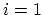and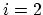, the overall transmission probability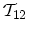between these two scattering centers is given by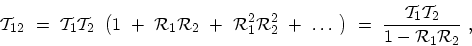(2.26)

where the effect of interference during a scattering event is neglected. One can rewrite (2.26) as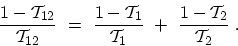(2.27)

Using the formula for the resistance given by (2.25) and (2.27), one can see that the resistance of the wire is additive,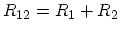. Applying this result to the case of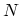scatterers yields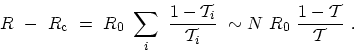(2.28)

where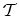is an average transmission probability for an individual scattering event over a mean free path. Thus the total resistance is given by a series connection of microscopic resistances. This is nothing but OHM's law, according to which the microscopic resistance is proportional to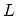. M. Pourfath: Numerical Study of Quantum Transport in Carbon Nanotube-Based Transistors